###### back to index | new

Point $B$ lies on line segment $\overline{AC}$ with $AB=16$ and $BC=4$. Points $D$ and $E$ lie on the same side of line $AC$ forming equilateral triangles $\triangle ABD$ and $\triangle BCE$. Let $M$ be the midpoint of $\overline{AE}$, and $N$ be the midpoint of $\overline{CD}$. The area of $\triangle BMN$ is $x$. Find $x^2$.

Point $A,B,C,D,$ and $E$ are equally spaced on a minor arc of a cirle. Points $E,F,G,H,I$ and $A$ are equally spaced on a minor arc of a second circle with center $C$ as shown in the figure below. The angle $\angle ABD$ exceeds $\angle AHG$ by $12^\circ$. Find the degree measure of $\angle BAG$.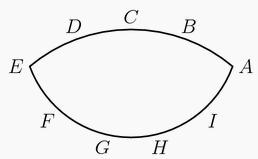Triangle $ABC$ has positive integer side lengths with $AB=AC$. Let $I$ be the intersection of the bisectors of $\angle B$ and $\angle C$. Suppose $BI=8$. Find the smallest possible perimeter of $\triangle ABC$.

Triangle $ABC$ has side lengths $AB = 12$, $BC = 25$, and $CA = 17$. Rectangle $PQRS$ has vertex $P$ on $\overline{AB}$, vertex $Q$ on $\overline{AC}$, and vertices $R$ and $S$ on $\overline{BC}$. In terms of the side length $PQ = w$, the area of $PQRS$ can be expressed as the quadratic polynomial Area($PQRS$) = $\alpha w - \beta \cdot w^2$. Then the coefficient $\beta = \frac{m}{n}$, where $m$ and $n$ are relatively prime positive integers. Find $m+n$.

The circumcircle of acute $\triangle ABC$ has center $O$. The line passing through point $O$ perpendicular to $\overline{OB}$ intersects lines $AB$ and $BC$ and $P$ and $Q$, respectively. Also $AB=5$, $BC=4$, $BQ=4.5$, and $BP=\frac{m}{n}$, where $m$ and $n$ are relatively prime positive integers. Find $m+n$.

Circles $\mathcal{P}$ and $\mathcal{Q}$ have radii $1$ and $4$, respectively, and are externally tangent at point $A$. Point $B$ is on $mathcal{P}$ and point $C$ is on $\mathcal{Q}$ so that line $BC$ is a common external tangent of the two circles. A line $\ell$ through $A$ intersects $\mathcal{P}$ again at $D$ and intersects $\mathcal{Q}$ again at $E$. Points $B$ and $C$ lie on the same side of $\ell$, and the areas of $\triangle DBA$ and $\triangle ACE$ are equal. This common area is $\frac{m}{n}$, where $m$ and $n$ are relatively prime positive integers. Find $m+n$.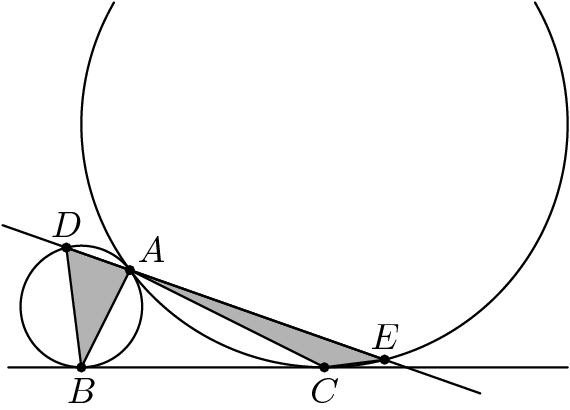A disk with radius $1$ is externally tangent to a disk with radius $5$. Let $A$ be the point where the disks are tangent, $C$ be the center of the smaller disk, and $E$ be the center of the larger disk. While the larger disk remains fixed, the smaller disk is allowed to roll along the outside of the larger disk until the smaller disk has turned through an angle of $360^\circ$. That is, if the center of the smaller disk has moved to the point $D$, and the point on the smaller disk that began at $A$ has now moved to point $B$, then $\overline{AC}$ is parallel to $\overline{BD}$. Then $sin^2(\angle BEA)=\tfrac{m}{n}$, where $m$ and $n$ are relatively prime positive integers. Find $m+n$.

On square $ABCD$, points $E,F,G$, and $H$ lie on sides $\overline{AB},\overline{BC},\overline{CD},$ and $\overline{DA},$ respectively, so that $\overline{EG} \perp \overline{FH}$ and $EG=FH = 34$. Segments $\overline{EG}$ and $\overline{FH}$ intersect at a point $P$, and the areas of the quadrilaterals $AEPH, BFPE, CGPF,$ and $DHPG$ are in the ratio $269:275:405:411.$ Find the area of square $ABCD$.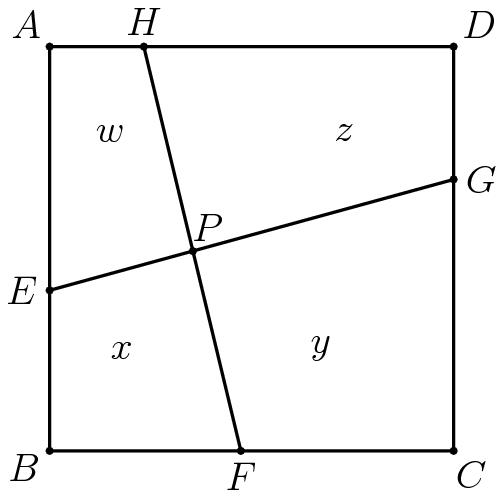A rectangle has sides of length $a$ and 36. A hinge is installed at each vertex of the rectangle, and at the midpoint of each side of length 36. The sides of length $a$ can be pressed toward each other keeping those two sides parallel so the rectangle becomes a convex hexagon as shown. When the figure is a hexagon with the sides of length $a$ parallel and separated by a distance of 24, the hexagon has the same area as the original rectangle. Find $a^2$.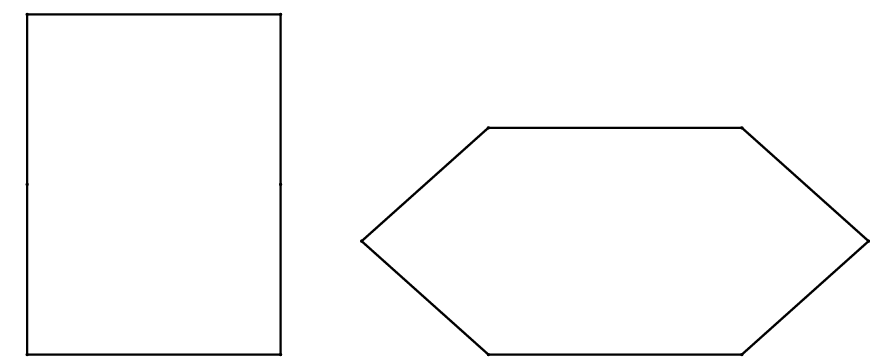Circle $C$ with radius 2 has diameter $\overline{AB}$. Circle D is internally tangent to circle $C$ at $A$. Circle $E$ is internally tangent to circle $C$, externally tangent to circle $D$, and tangent to $\overline{AB}$. The radius of circle $D$ is three times the radius of circle $E$, and can be written in the form $\sqrt{m}-n$, where $m$ and $n$ are positive integers. Find $m+n$.

In $\triangle RED$, $\angle DRE=75^{\circ}$ and $\angle RED=45^{\circ}$. $|RD|=1$. Let $M$ be the midpoint of segment $\overline{RD}$. Point $C$ lies on side $\overline{ED}$ such that $\overline{RC}\perp\overline{EM}$. Extend segment $\overline{DE}$ through $E$ to point $A$ such that $CA=AR$. Then $AE=\frac{a-\sqrt{b}}{c}$, where $a$ and $c$ are relatively prime positive integers, and $b$ is a positive integer. Find $a+b+c$.

In $\triangle{ABC}, AB=10, \angle{A}=30^{\circ}$, and $\angle{C=45^{\circ}}$. Let $H, D,$ and $M$ be points on the line $BC$ such that $AH\perp{BC}$, $\angle{BAD}=\angle{CAD}$, and $BM=CM$. Point $N$ is the midpoint of the segment $HM$, and point $P$ is on ray $AD$ such that $PN\perp{BC}$. Then $AP^2=\dfrac{m}{n}$, where $m$ and $n$ are relatively prime positive integers. Find $m+n$.

Let $ABCD$ be a square, and let $E$ and $F$ be points on $\overline{AB}$ and $\overline{BC},$ respectively. The line through $E$ parallel to $\overline{BC}$ and the line through $F$ parallel to $\overline{AB}$ divide $ABCD$ into two squares and two nonsquare rectangles. The sum of the areas of the two squares is $\frac{9}{10}$ of the area of square $ABCD.$ Find $\frac{AE}{EB} + \frac{EB}{AE}.$

A paper equilateral triangle $ABC$ has side length $12$. The paper triangle is folded so that vertex $A$ touches a point on side $\overline{BC}$ a distance $9$ from point $B$. The length of the line segment along which the triangle is folded can be written as $\frac{m\sqrt{p}}{n}$, where $m$, $n$, and $p$ are positive integers, $m$ and $n$ are relatively prime, and $p$ is not divisible by the square of any prime. Find $m+n+p$.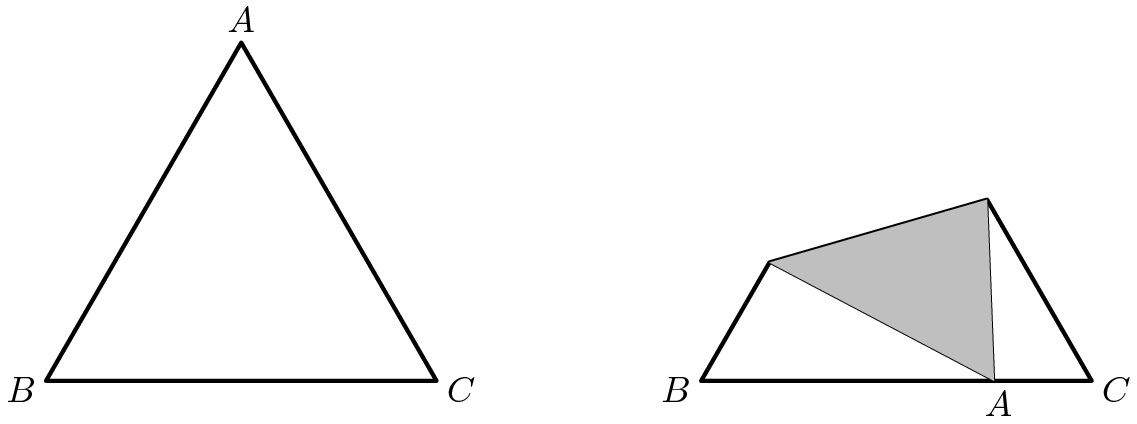Let $\bigtriangleup PQR$ be a triangle with $\angle P = 75^\circ$ and $\angle Q = 60^\circ$. A regular hexagon $ABCDEF$ with side length 1 is drawn inside $\triangle PQR$ so that side $\overline{AB}$ lies on $\overline{PQ}$, side $\overline{CD}$ lies on $\overline{QR}$, and one of the remaining vertices lies on $\overline{RP}$. There are positive integers $a, b, c,$ and $d$ such that the area of $\triangle PQR$ can be expressed in the form $\frac{a+b\sqrt{c}}{d}$, where $a$ and $d$ are relatively prime, and c is not divisible by the square of any prime. Find $a+b+c+d$.

Triangle $AB_0C_0$ has side lengths $AB_0 = 12$, $B_0C_0 = 17$, and $C_0A = 25$. For each positive integer $n$, points $B_n$ and $C_n$ are located on $\overline{AB_{n-1}}$ and $\overline{AC_{n-1}}$, respectively, creating three similar triangles $\triangle AB_nC_n \sim \triangle B_{n-1}C_nC_{n-1} \sim \triangle AB_{n-1}C_{n-1}$. The area of the union of all triangles $B_{n-1}C_nB_n$ for $n\geq1$ can be expressed as $\tfrac pq$, where $p$ and $q$ are relatively prime positive integers. Find $q$.

In equilateral $\triangle ABC$ let points $D$ and $E$ trisect $\overline{BC}$. Then $\sin(\angle DAE)$ can be expressed in the form $\frac{a\sqrt{b}}{c}$, where $a$ and $c$ are relatively prime positive integers, and $b$ is an integer that is not divisible by the square of any prime. Find $a+b+c$.

A hexagon that is inscribed in a circle has side lengths $22$, $22$, $20$, $22$, $22$, and $20$ in that order. The radius of the circle can be written as $p+\sqrt{q}$, where $p$ and $q$ are positive integers. Find $p+q$.

Given a circle of radius $\sqrt{13}$, let $A$ be a point at a distance $4 + \sqrt{13}$ from the center $O$ of the circle. Let $B$ be the point on the circle nearest to point $A$. A line passing through the point $A$ intersects the circle at points $K$ and $L$. The maximum possible area for $\triangle BKL$ can be written in the form $\frac{a - b\sqrt{c}}{d}$, where $a$, $b$, $c$, and $d$ are positive integers, $a$ and $d$ are relatively prime, and $c$ is not divisible by the square of any prime. Find $a+b+c+d$.

In $\triangle ABC$, $AC = BC$, and point $D$ is on $\overline{BC}$ so that $CD = 3\cdot BD$. Let $E$ be the midpoint of $\overline{AD}$. Given that $CE = \sqrt{7}$ and $BE = 3$, the area of $\triangle ABC$ can be expressed in the form $m\sqrt{n}$, where $m$ and $n$ are positive integers and $n$ is not divisible by the square of any prime. Find $m+n$.

Let $\triangle ABC$ be a right triangle with right angle at $C.$ Let $D$ and $E$ be points on $\overline{AB}$ with $D$ between $A$ and $E$ such that $\overline{CD}$ and $\overline{CE}$ trisect $\angle C.$ If $\frac{DE}{BE} = \frac{8}{15},$ then $\tan B$ can be written as $\frac{m \sqrt{p}}{n},$ where $m$ and $n$ are relatively prime positive integers, and $p$ is a positive integer not divisible by the square of any prime. Find $m+n+p.$

Three concentric circles have radii $3,$ $4,$ and $5.$ An equilateral triangle with one vertex on each circle has side length $s.$ The largest possible area of the triangle can be written as $a + \tfrac{b}{c} \sqrt{d},$ where $a,$ $b,$ $c,$ and $d$ are positive integers, $b$ and $c$ are relatively prime, and $d$ is not divisible by the square of any prime. Find $a+b+c+d.$

Equilateral $\triangle ABC$ has side length $\sqrt{111}$. There are four distinct triangles $AD_1E_1$, $AD_1E_2$, $AD_2E_3$, and $AD_2E_4$, each congruent to $\triangle ABC$, with $BD_1 = BD_2 = \sqrt{11}$. Find $\displaystyle\sum_{k=1}^4(CE_k)^2$.

Triangle $ABC$ is inscribed in circle $\omega$ with $AB=5$, $BC=7$, and $AC=3$. The bisector of angle $A$ meets side $\overline{BC}$ at $D$ and circle $\omega$ at a second point $E$. Let $\gamma$ be the circle with diameter $\overline{DE}$. Circles $\omega$ and $\gamma$ meet at $E$ and a second point $F$. Then $AF^2 = \frac mn$, where $m$ and $n$ are relatively prime positive integers. Find $m+n$.

In rectangle $ABCD$, $AB=12$ and $BC=10$. Points $E$ and $F$ lie inside rectangle $ABCD$ so that $BE=9$,$DF=8$,$\overline{BE}||\overline{DF}$,$\overline{EF}||\overline{AB}$, and line $BE$ intersects segment $\overline{AD}$. The length $EF$ can be expressed in the form $m\sqrt{n}-p$, where $m$,$n$, and $p$ are positive integers and $n$ is not divisible by the square of any prime. Find $m+n+p$.